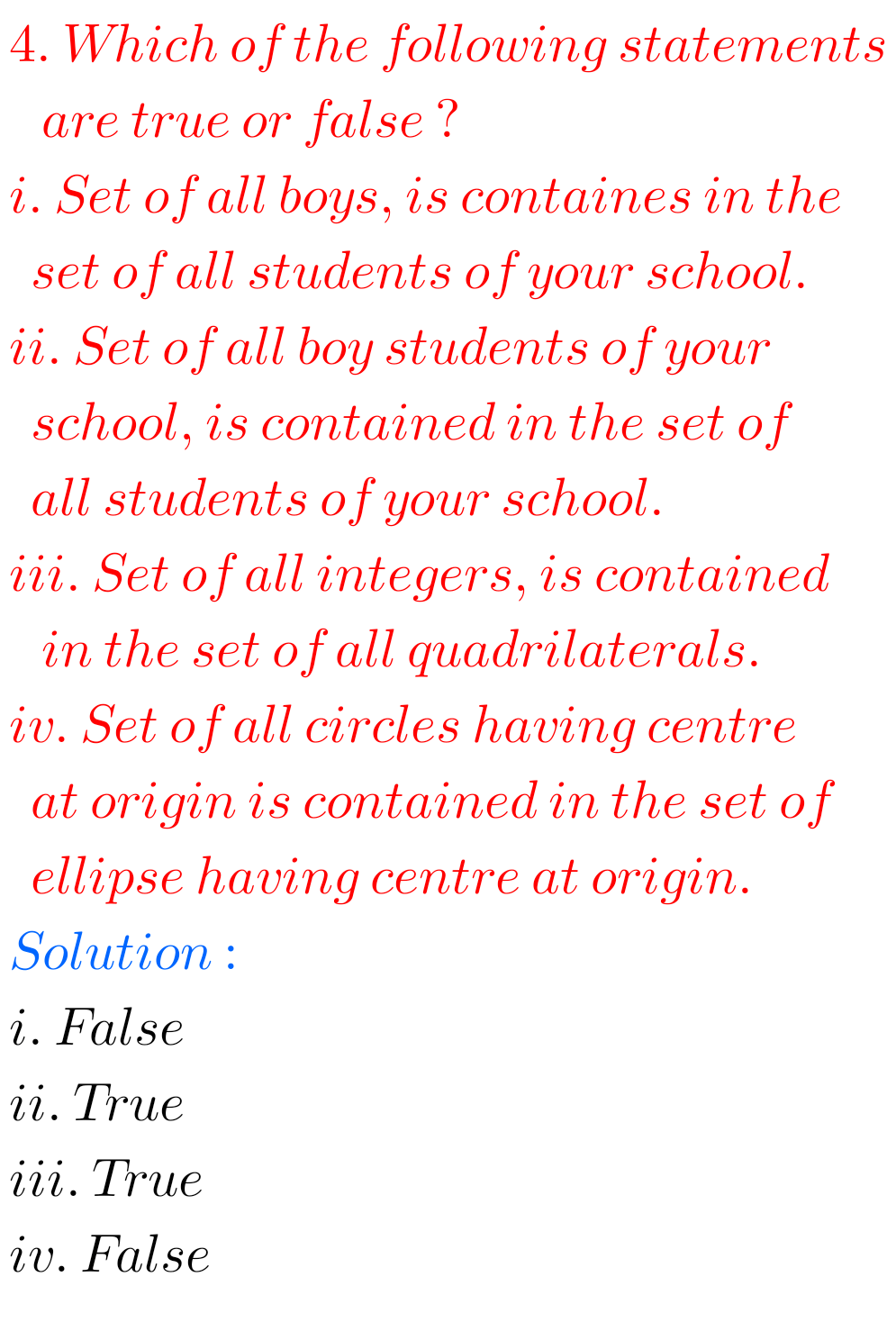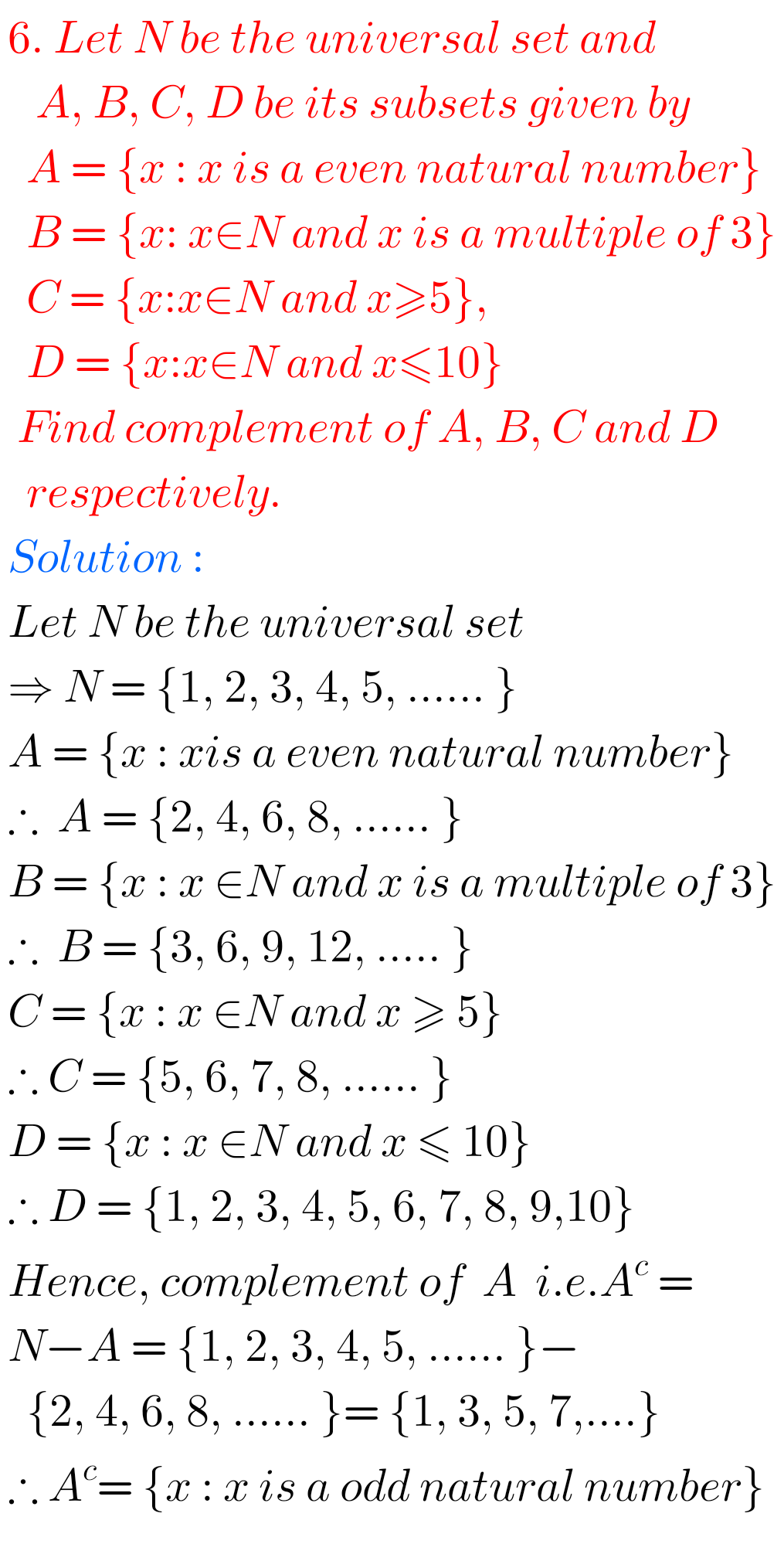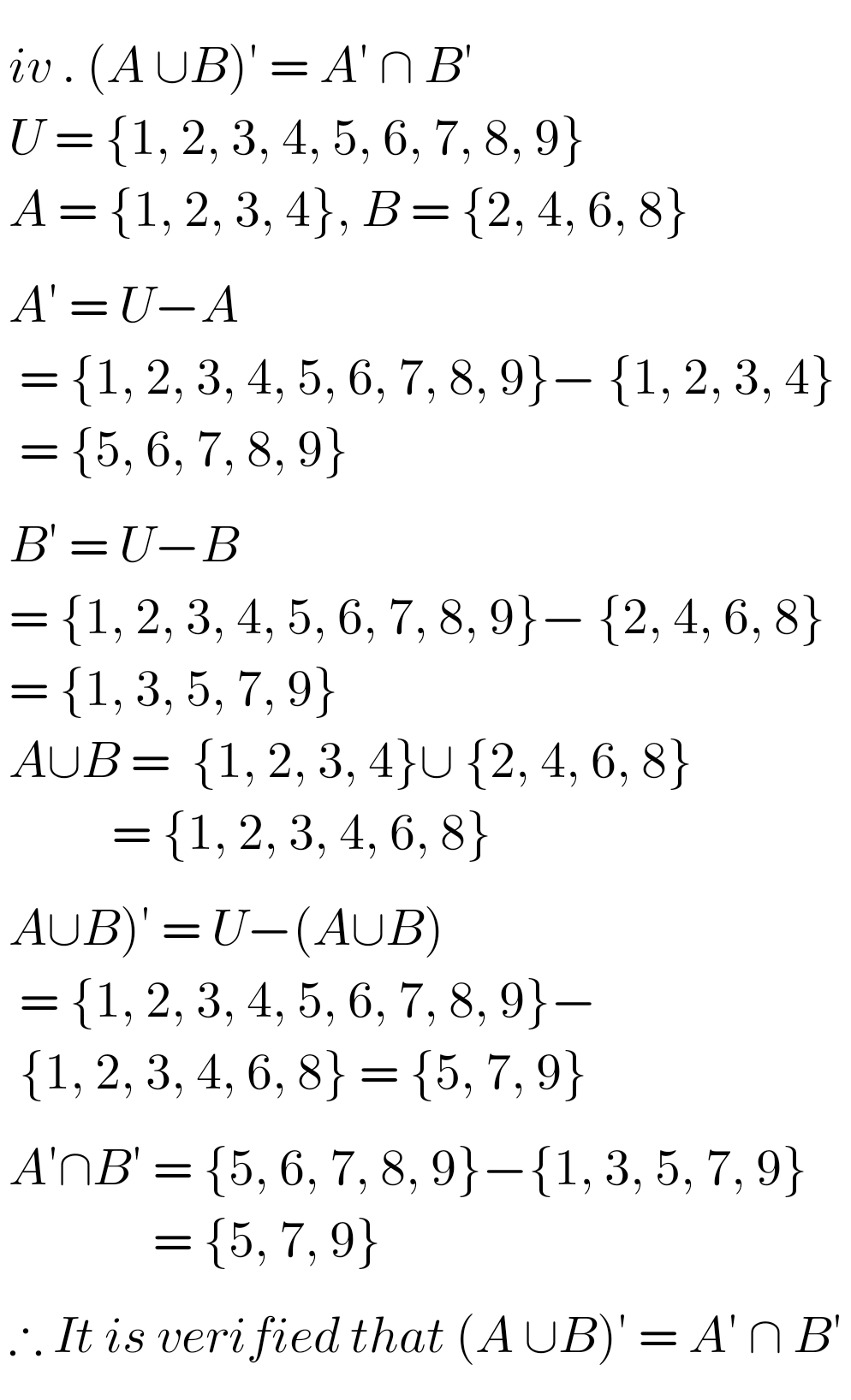# Sets solutions for check your progress 1.2 NIOS Maths 311 book 1

Senior secondary Maths 311 book 1 Solutions for Sets check your progress 1.2 are given.

You should study the textbook lesson Sets very well. Observe the example problem and solutions given in the textbook.

Try the example problems and solutions given in the textbook NIOS.

Observe the given below solutions and try them in your own method.

You can see solutions for NIOS Maths 311 book.

1. Sets

NIOS Maths 311 book 2 Solutions for some chapters

# Senior secondary solutions for maths book 1 Sets 1.2 NIOS

Sets solutions check your progress 1.2 maths 311 book 1 NIOSM

## Maths 311 solutions for Sets 1.2 book 1 NIOS## Solutions for Sets 1.2 NIOS Maths 311M

Note : Observe the solutions and try them in your own method.

SSC Maths Solutions class 10 AP and TS

Inter Maths Solutions 1A

Inter Maths Solutions IIA Quadratic expressions

NIOS Maths 311 book 2 Solutions for some chapters

NCERT class 6 maths solutions

NCERT class 7 maths solutions

Inter maths solutions

SSC maths Solutions class 10